MATLAB Function Referencelegendre

Associated Legendre functions

Syntax

• ```P` `=` `legendre(n,X)
S = legendre(n,X,'sch')
N = legendre(n,X,'norm')
```

Definitions

Associated Legendre Functions.   The Legendre functions are defined by

•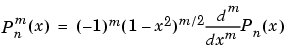where

•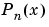is the Legendre polynomial of degree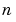.

•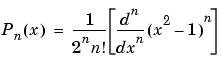Schmidt Seminormalized Associated Legendre Functions.   The Schmidt seminormalized associated Legendre functions are related to the nonnormalized associated Legendre functions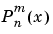by

•` `for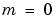•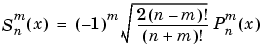` ` for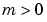.

Fully Normalized Associated Legendre Functions.   The fully normalized associated Legendre functions are normalized such that

•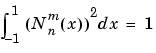and are related to the unnormalized associated Legendre functionsby

•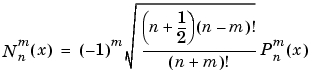Description

```P = legendre(n,X) ``` computes the associated Legendre functions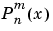of degree `n` and order `m = 0,1,...,n`, evaluated for each element of `X`. Argument `n` must be a scalar integer, and `X` must contain real values in the domain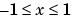.

If `X` is a vector, then `P` is an `(n+1)`-by-`q` matrix, where `q = length(X)`. Each element `P(m+1,i)` corresponds to the associated Legendre function of degree `n` and order `m` evaluated at `X(i)`.

In general, the returned array `P` has one more dimension than `X`, and each element `P(m+1,i,j,k,...)` contains the associated Legendre function of degree `n` and order `m` evaluated at `X(i,j,k,...)`. Note that the first row of `P` is the Legendre polynomial evaluated at `X`, i.e., the case where `m` = 0.

```S = legendre(n,X,'sch') ``` computes the Schmidt seminormalized associated Legendre functions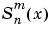.

```N = legendre(n,X,'norm') ``` computes the fully normalized associated Legendre functions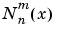.

Examples

Example 1. The statement `legendre(2,0:0.1:0.2)` returns the matrix

 x = 0 x = 0.1 x = 0.2 m = 0 `-0.5000` `-0.4850` `-0.4400` m = 1 ` 0` `-0.2985` `-0.5879` m = 2 ` 3.0000` ` 2.9700` ` 2.8800`

Example 2. Given,

• ```X = rand(2,4,5);
n = 2;
P = legendre(n,X)
```

then

• ```size(P)
ans =
3     2     4     5
```

and

• ```P(:,1,2,3)
ans =
-0.2475
-1.1225
2.4950
```

is the same as

• ```legendre(n,X(1,2,3))
ans =
-0.2475
-1.1225
2.4950
```

Algorithm

`legendre` uses a three-term backward recursion relationship in `m`. This recursion is on a version of the Schmidt seminormalized associated Legendre functions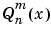, which are complex spherical harmonics. These functions are related to the standard Abramowitz and Stegun  functionsby

•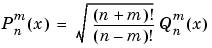They are related to the Schmidt form given previously by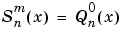` ` for•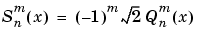` `for.

References

  Abramowitz, M. and I. A. Stegun, Handbook of Mathematical Functions, Dover Publications, 1965, Ch.8.

  Jacobs, J. A., Geomagnetism, Academic Press, 1987, Ch.4.

© 1994-2005 The MathWorks, Inc.